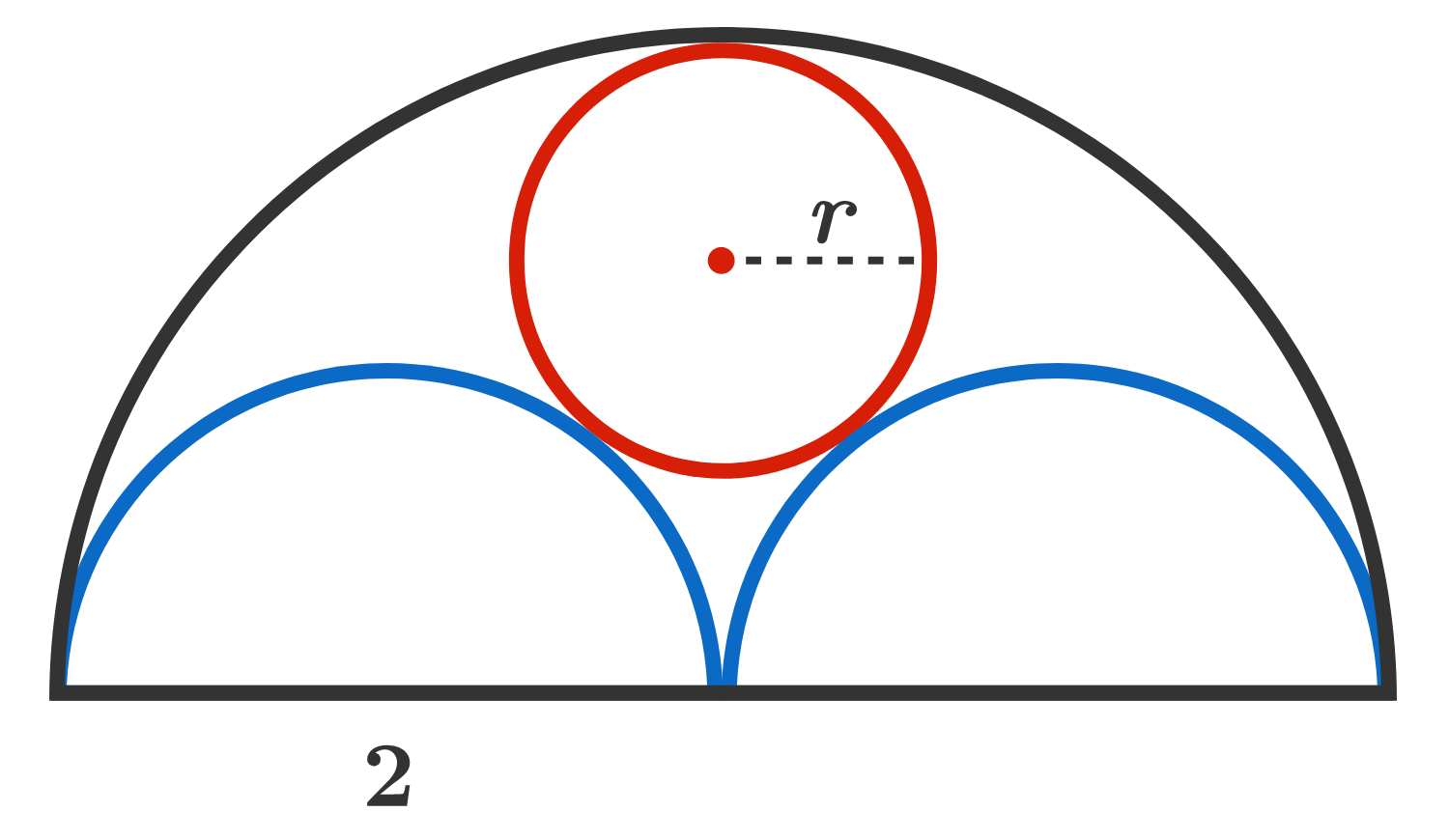# Circle Geometry Question

Geometry Level 2

In the figure below, there is a semicircle with radius 2 and two identical semicircles within it, each with radius 1. The smaller semicircles are externally tangent to each other and share the edge with the larger semicircle. There is also a circle tangent to all three semicircles (internally tangent to the large semicircle, and externally tangent to the smaller semicircles).What is the radius of the circle?

×